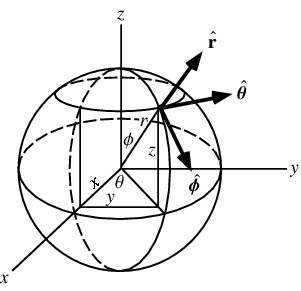# Spherical Coordinates

## Homework Statement

Set up the triple integral for the volume of the given solid using spherical coordinates:

The solid bounded below by the sphere ρ=6cosθ and above by the cone z=sqrt(x2+y2)

## The Attempt at a Solution

I thought i had this set up right where ρ goes from 6cosθ to sqrt(3)/2 ( how i got sqrt(3)/2 was by z=sqrt(x2+y2 is th esame as ρcos∅=ρsin which means ∅=pi/4 plugging that into ρ=6cosθ, gives me sqrt3/2) i don't know if this is right but for ∅ it goes from 0 to pi/4 and for θ it goes from 0 to 2pi

ehild
Homework Helper
What is ρ, and what is θ?

How can be ρ=6cosθ the equation of a sphere?

ehild

ρ is the distance from P to the origin

∅ is the angle that the line makes with the positive x axis

θ is the angle from the cylindrical coordinates (0<θ<2pi)

i know that the equation for a sphere is when ρ= constant, in this case she told us it was cos∅

ehild
Homework Helper
Could you please show that sphere and cone and the volume you need to find, placed into the system of coordinates you use? Is that the same as in the picture? (From http://mathworld.wolfram.com/SphericalCoordinates.html)ehild

Last edited:
ehild
Homework Helper
is that the volume you nee to find?

http://img811.imageshack.us/img811/4920/conesphere.jpg [Broken]

ehild

Last edited by a moderator:
i couldnt get a picture but that is how the sphere looks with a radius of six and the cone is just the top half as looking as any cone would look

ehild
Homework Helper
Is the volume as shown in the picture in my previous post? If so, you have to integrate the volume element dV=r^2sin(∅)dr d∅ dθ from 0 to 2pi for θ,
from 0 to pi/4 for ∅ (it is the angle of the cone it makes with the positive y axis) and for r from the top of the cone to the radius of the sphere r=ρ=6.

ehild